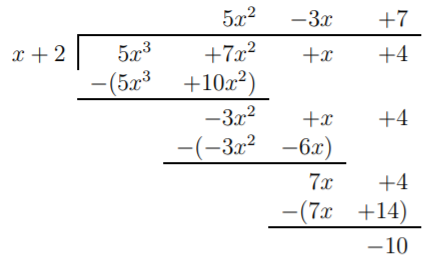# 8.3: Optional section- Synthetic division

$$\newcommand{\vecs}{\overset { \rightharpoonup} {\mathbf{#1}} }$$ $$\newcommand{\vecd}{\overset{-\!-\!\rightharpoonup}{\vphantom{a}\smash {#1}}}$$$$\newcommand{\id}{\mathrm{id}}$$ $$\newcommand{\Span}{\mathrm{span}}$$ $$\newcommand{\kernel}{\mathrm{null}\,}$$ $$\newcommand{\range}{\mathrm{range}\,}$$ $$\newcommand{\RealPart}{\mathrm{Re}}$$ $$\newcommand{\ImaginaryPart}{\mathrm{Im}}$$ $$\newcommand{\Argument}{\mathrm{Arg}}$$ $$\newcommand{\norm}{\| #1 \|}$$ $$\newcommand{\inner}{\langle #1, #2 \rangle}$$ $$\newcommand{\Span}{\mathrm{span}}$$ $$\newcommand{\id}{\mathrm{id}}$$ $$\newcommand{\Span}{\mathrm{span}}$$ $$\newcommand{\kernel}{\mathrm{null}\,}$$ $$\newcommand{\range}{\mathrm{range}\,}$$ $$\newcommand{\RealPart}{\mathrm{Re}}$$ $$\newcommand{\ImaginaryPart}{\mathrm{Im}}$$ $$\newcommand{\Argument}{\mathrm{Arg}}$$ $$\newcommand{\norm}{\| #1 \|}$$ $$\newcommand{\inner}{\langle #1, #2 \rangle}$$ $$\newcommand{\Span}{\mathrm{span}}$$$$\newcommand{\AA}{\unicode[.8,0]{x212B}}$$

When dividing a polynomial $$f(x)$$ by $$g(x)=x-c$$, the actual calculation of the long division has a lot of unnecessary repetitions, and we may want to reduce this redundancy as much as possible. In fact, we can extract the essential part of the long division, the result of which is called synthetic division.

## Example $$\PageIndex{1}$$

Our first example is the long division of $$\dfrac{5x^3+7x^2+x+4}{x+2}$$.

SolutionHere, the first term $$5x^2$$ of the quotient is just copied from the first term of the dividend. We record this together with the coefficients of the dividend $$5x^3+7x^2+x+4$$ and of the divisor $$x+2=x-(-2)$$ as follows:

$\begin{array}{c|cccc} & 5 & 7 & 1 & 4 \qquad \text{ (dividend)}(5 x^{3}+7 x^{2}+x+4)\\ -2 &&&& \text{ (divisor)}(x-(-2))\\ \hline\\ & 5 &&& \text{ (quotient)}\\ \end{array} \nonumber$

The first actual calculation is performed when multiplying the $$5x^2$$ term with $$2$$, and subtracting it from $$7x^2$$. We record this as follows.Similarly, we obtain the next step by multiplying the $$2x$$ by $$(-3)$$ and subtracting it from $$1x$$. Therefore, we get:The last step multiplies $$7$$ times $$2$$ and subtracts this from $$4$$. In short, we write:The answer can be determined from these coefficients. The quotient is $$5x^2-3x+7$$, and the remainder is $$-10$$.

## Example $$\PageIndex{2}$$

Find the following quotients via synthetic division.

1. $$\dfrac{4x^3-7x^2+4x-8}{x-4}$$
2. $$\dfrac{x^4-x^2+5}{x+3}$$

Solution

1. We need to perform the synthetic division. $\begin{array}{c|cccc} & 4 & -7 & 4 & -8 \\ 4 && 16 & 36 & 160 \\ \hline\\ & 4 & 9 & 40 & 152\\ \end{array} \nonumber$

Therefore we have

$\dfrac{4x^3-7x^2+4x-8}{x-4}=4x^2+9x+40+\dfrac{152}{x-4} \nonumber$

2. Similarly, we calculate part (b). Note that some of the coefficients are now zero. $\begin{array}{c|ccccc} & 1 & \quad 0 & -1 & \quad 0 & 5 \\ -3 && -3 & \quad 9 & -24 & 72 \\ \hline\\ & 1 & -3 & 8 & -24 & 77\\ \end{array} \nonumber$

We obtain the following result:

$\dfrac{x^4-x^2+5}{x+3}=x^3-3x^2+8x-24+\dfrac{77}{x+5} \nonumber$

## Note

Synthetic division only works when dividing by a polynomial of the form $$x-c$$. Do not attempt to use this method to divide by other forms like $$x^2+2$$.

This page titled 8.3: Optional section- Synthetic division is shared under a CC BY-NC-SA 4.0 license and was authored, remixed, and/or curated by Thomas Tradler and Holly Carley (New York City College of Technology at CUNY Academic Works) via source content that was edited to the style and standards of the LibreTexts platform; a detailed edit history is available upon request.